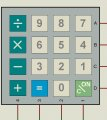# Need help on Matrix Keypad Interface Logic

#### Parth786

Joined Jun 19, 2017
642
I am looking help on matrix keypad. I searched on internet and having difficulty to understand logic of matrix keypad. Matrix keypad use a combination of four rows and four columns to provide button states to the device ( microcontroller). Each key is a push button, with one end connected to one row, and the other end connected to one column.this is component from Proteus. I was looking datasheet but I couldn't found. How does keypad work with microcontroller. what is basic logic of Matrix keypad?

#### Alec_t

Joined Sep 17, 2013
14,005
The micro writes a given logic level to each column (or row) in turn and, while the written-to column (or row) is held at that logic level, reads each row (or column) in turn to determine which one or more has that logic level.

#### Sensacell

Joined Jun 19, 2012
3,327
It's a scanning process, the switches are read row-by-row.

Energize one row, read the state of the 4 columns, then do the next one.
Reads 16 switches with one 8 bit port.

#### Parth786

Joined Jun 19, 2017
642
any help with example. By seeing definition only its difficult for me to understand operation of keypad. there are 8 inputs connected to microcontroller. 4 column inputs and 4 row inputs My basic confusion is that I don't understand on which logic this keypad work?

#### EM Fields

Joined Jun 8, 2016
583
any help with example. By seeing definition only its difficult for me to understand operation of keypad. there are 8 inputs connected to microcontroller. 4 column inputs and 4 row inputs My basic confusion is that I don't understand on which logic this keypad work?

#### Picbuster

Joined Dec 2, 2013
1,045
Attached an example. Next thing to do is to grab a PIC and write the codes to read matrix.
Picbuster

#### Attachments

• 34.8 KB Views: 9

#### LesJones

Joined Jan 8, 2017
4,101
You need 4 bits of one port set as outputs driving say the X axis of the keyboard and 4 bits of another port configured as inputs looking ast the 4 bits from the Y axis.You set one bit of the X axis high the read the Y axis and see which bit is high. If you see a high on any of the 4 bits then you know the button that was pressed. You then repeat the process with the other 3 bits on the X axis high in turn. You will then have tested all 16 keys. With the logic working the way round as I described it you will need pull down resistors on the logic inputs.

Les.

•EM Fields

Joined Jul 18, 2013
28,020
You need 4 bits of one port set as outputs driving say the X axis of the keyboard and 4 bits of another port configured as inputs looking ast the 4 bits from the Y axis.
Les.
... Or you can use one port for both as in the N.Goodwin example.Max.

#### Parth786

Joined Jun 19, 2017
642
You need 4 bits of one port set as outputs driving say the X axis of the keyboard and 4 bits of another port configured as inputs looking ast the 4 bits from the Y axis.You set one bit of the X axis high the read the Y axis and see which bit is high. If you see a high on any of the 4 bits then you know the button that was pressed. You then repeat the process with the other 3 bits on the X axis high in turn. You will then have tested all 16 keys. With the logic working the way round as I described it you will need pull down resistors on the logic inputs.

Les.
I am referring my diagram.
did you mean like this

X port as output 1234
Y port as input ABCD

set one bit of the X axis high the read the Y axis and see which bit is high
if bit 1 is high

1=1 2=0 3=0 4=0

A=1 B= C=0 D=0---------- How to know that which button was pressed
A=0 B=1 C=0 D=0-------- How to know that which button was pressed
A=0 B=0 C=1 D=0---------How to know that which button was pressed
A=0 B=0 C=0 D=1---------How to know that which button was pressed

if bit 2 is high
1=0 2=1 3=0 4=0

A=1 B= C=0 D=0----------How to know that which button was pressed
A=0 B=1 C=0 D=0--------How to know that which button was pressed
A=0 B=0 C=1 D=0------- How to know that which button was pressed
A=0 B=0 C=0 D=1---------How to know that which button was pressed

how to know which button was pressed ?